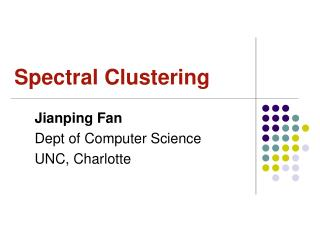DownloadDownload PresentationSpectral Clustering

# Spectral Clustering

Télécharger la présentation## Spectral Clustering

- - - - - - - - - - - - - - - - - - - - - - - - - - - E N D - - - - - - - - - - - - - - - - - - - - - - - - - - -
##### Presentation Transcript

1. Spectral Clustering Jianping Fan Dept of Computer Science UNC, Charlotte

2. Lecture Outline • Motivation • Graph overview and construction • Spectral Clustering • Cool implementations

3. Semantic interpretations of clustering clusters

4. Dataset exhibits complex cluster shapes • K-means performs very poorly in this space due bias toward dense spherical clusters. In the embedded space given by two leading eigenvectors, clusters are trivial to separate. Spectral Clustering Example – 2 Spirals

5. Spectral Clustering Example Original Points K-means (2 Clusters) Why k-means fail for these two examples?

6. Lecture Outline • Motivation • Graph overview and construction • Spectral Clustering • Cool implementation

7. Graph-based Representation of Data Similarity

8. similarity Graph-based Representation of Data Similarity

9. Graph-based Representation of Data Relationship

10. Manifold

11. Graph-based Representation of Data Relationships

12. Data Graph Construction

13. Graph-based Representation of Data Relationships

14. Graph-based Representation of Data Relationships

15. Graph-based Representation of Data Relationships

16. Graph-based Representation of Data Relationships

17. Graph Cut

18. Lecture Outline • Motivation • Graph overview and construction • Spectral Clustering • Cool implementations

19. Graph-based Representation of Data Relationships

20. Graph Cut

21. Graph-based Representation of Data Relationships

22. Graph Cut

23. Eigenvectors & Eigenvalues

24. Normalized Cut A graph G(V, E) can be partitioned into two disjoint sets A, B Cut is defined as: Optimal partition of the graph G is achieved by minimizing the cut Min ( )

25. Normalized Cut Normalized Cut Association between partition set and whole graph

26. Normalized Cut

27. Normalized Cut

28. Normalized Cut

29. Normalized Cut Normalized Cut becomes Normalized cut can be solved by eigenvalue equation:

30. K-way Min-Max Cut Intra-cluster similarity Inter-cluster similarity Decision function for spectral clustering

31. Mathematical Description of Spectral Clustering Refined decision function for spectral clustering We can further define:

32. Refined decision function for spectral clustering This decision function can be solved as

33. Spectral Clustering Algorithm Ng, Jordan, and Weiss • Motivation • Given a set of points • We would like to cluster them into k subsets

34. Algorithm • Form the affinity matrix • Define if • Scaling parameter chosen by user • Define D a diagonal matrix whose (i,i) element is the sum of A’s row i

35. Algorithm • Form the matrix • Find , the k largest eigenvectors of L • These form the the columns of the new matrix X • Note: have reduced dimension from nxn to nxk

36. Algorithm • Form the matrix Y • Renormalize each of X’s rows to have unit length • Y • Treat each row of Y as a point in • Cluster into k clusters via K-means

37. Algorithm • Final Cluster Assignment • Assign point to cluster j iff row i of Y was assigned to cluster j